# Topic 9: Wave phenomena (HL)

See the guide for this topic.

## 9.1 – Simple harmonic motion

• ### The defining equation of SHM

By Newton’s Second Law, SHM can be defined as the following equations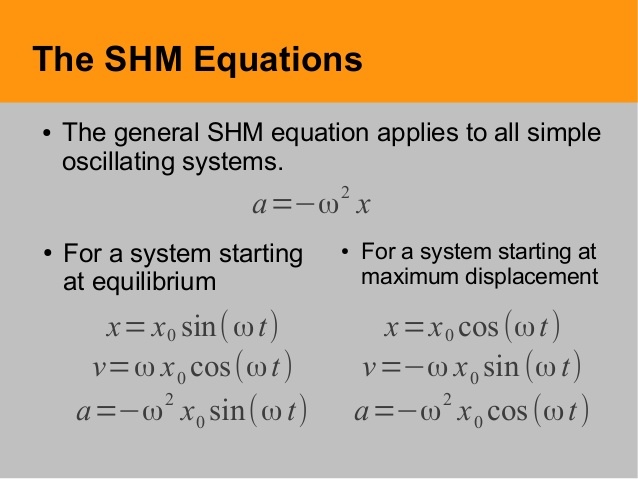where x0 is the amplitude (maximum displacement), x is the displacement, v is the velocity, and a is the acceleration.

The angular frequency ( w ) is related to the period of the SHM by the following equation• ### Energy changes

In a SHM, there is an interchange between KE and PE throughout the motion. However, the total energy remains constant.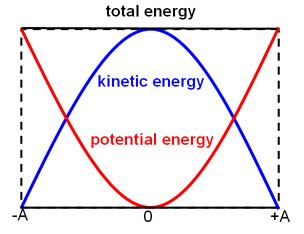Summary:

• At maximum displacement, PE is at max while KE=0
• At zero displacement, KE is at max while PE=0
• At minimum displacement, PE is at max while KE=0
• Total energy (KE+PE) remains constant throughout the motion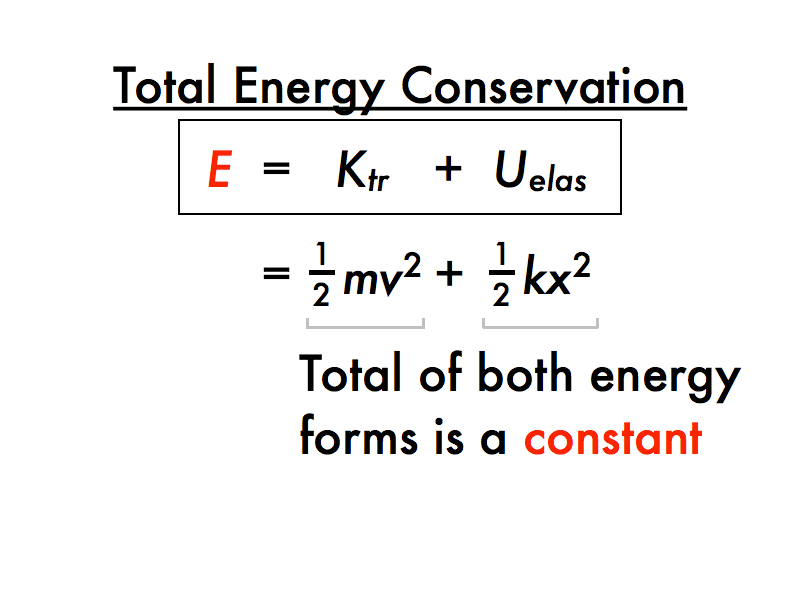## 9.2 – Single-slit diffraction

• ### The nature of single-slit diffraction

Special diffraction patterns appear when light is diffracted by a single slit which is comparable to the wavelength of the light in size.

We can represent this diffraction pattern by plotting the light intensity against the angle of diffraction.

The angle of diffraction for the first minimum θ can be given by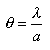where λ is the wavelength and a is the size/length of the slit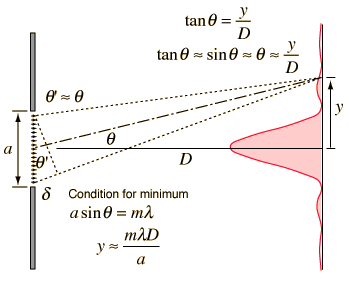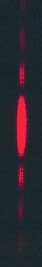where λ is the wavelength, m is the order of the maximum, D is the distance of the slits to the screen, and a is width of the slit.

## 9.3 – Interference

• ### Young’s double-slit experimentwhere λ is the wavelength, m is the order of the maximum, D is the distance of the slits to the screen, and d is the distance between the two slits.

• ### Modulation of two-slit interference pattern by one-slit diffraction effect

The previously section shows an ideal double-slit which ignores the single-slit characteristics of each of the two single-slits. A true double-slit would exhibit closely spaced dark and light areas (fringes) superimposed over the single-slit pattern. The single-slit profile is said to modulate the double-slit pattern.• ### Multiple slit and diffraction grating interference patterns

• Multiple slit interference patterns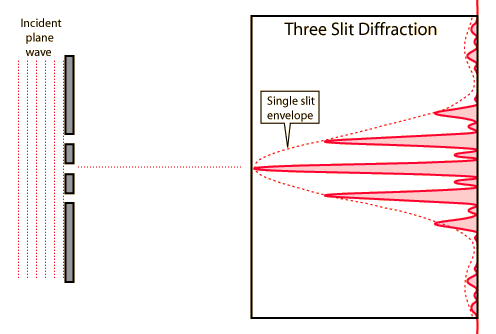• Diffraction grating interference patterns

A diffraction grating is the tool of choice for separating the colors in incident light.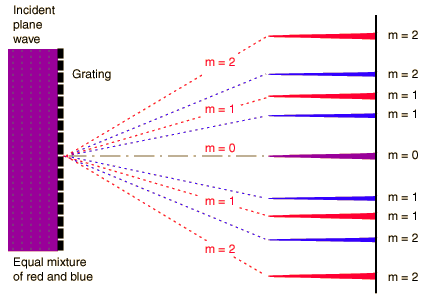The condition for maximum intensity is the same as that for a double-slit. However, the angular separation of the maxima is generally much greater because the slit spacing is so small for a diffraction grating.

The equation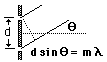is the condition for angles at which constructive interference occurs (maximum) where d is the distance between gratings and m is the order of the maximum.• ### Thin film interference

Interference between light waves is the reason that thin films, such as soap bubbles, show colorful patterns.

The interference of light waves reflects off the top surface of a film with the waves reflecting from the bottom of the surface.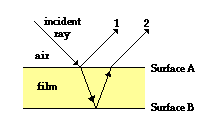## 9.4 – Resolution

• ### The size of a diffracting aperture

When light from a point source passes through a small circular aperture, it does not produce a bright dot as an image, but rather as a diffused circular disc.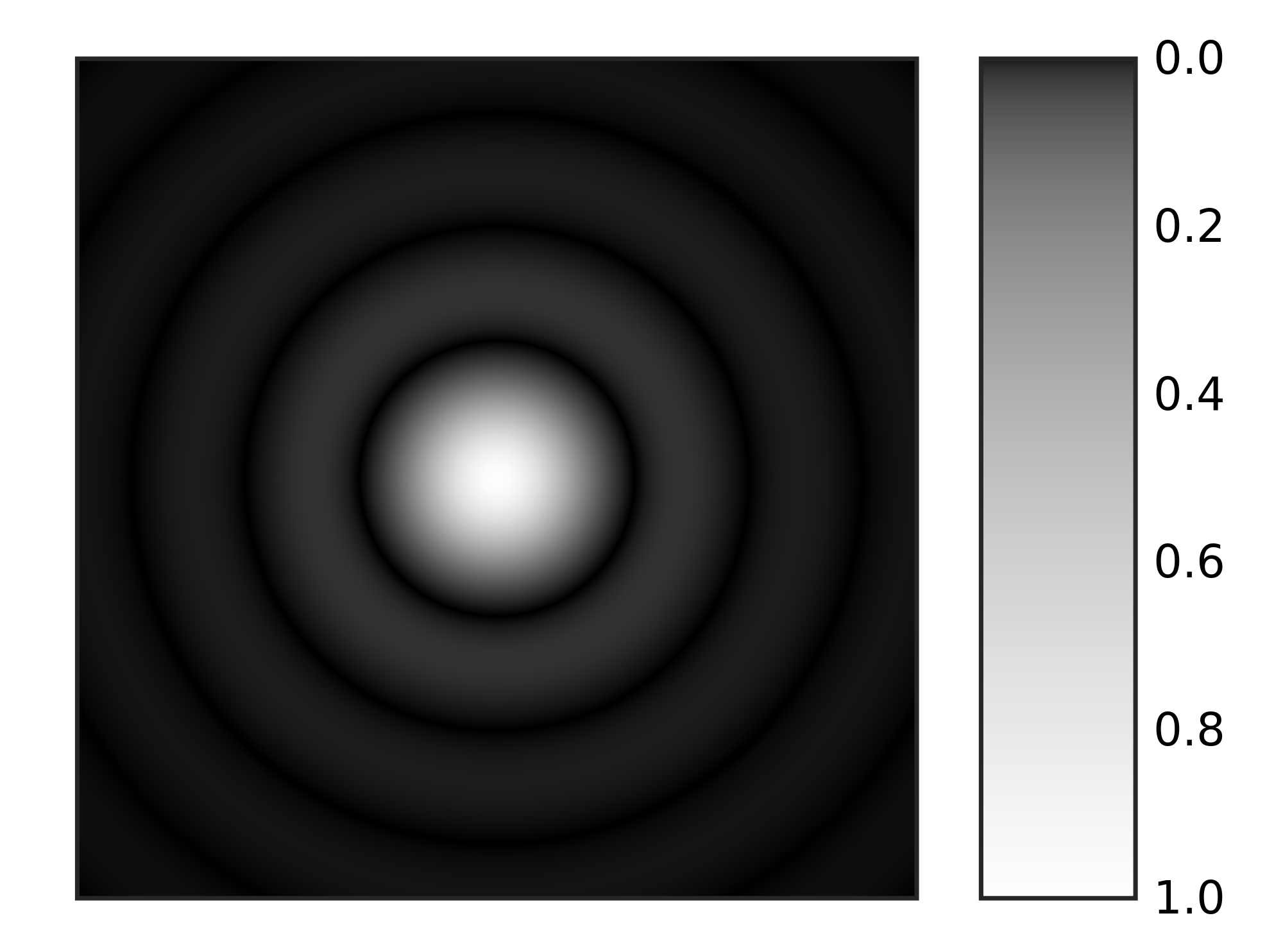The greater the diameter of the diffracting aperture (such as the diameter of the pupil in the human eye or the diameter of the lens in a telescope), the better resolved (clearer) the image is.

• ### The resolution of simple monochromatic two-source systems

Consider the diffraction pattern of two light beams diffracted by a single slit. These patterns can be categorized as resolved, just resolved, or not resolved depending on the separation between the images.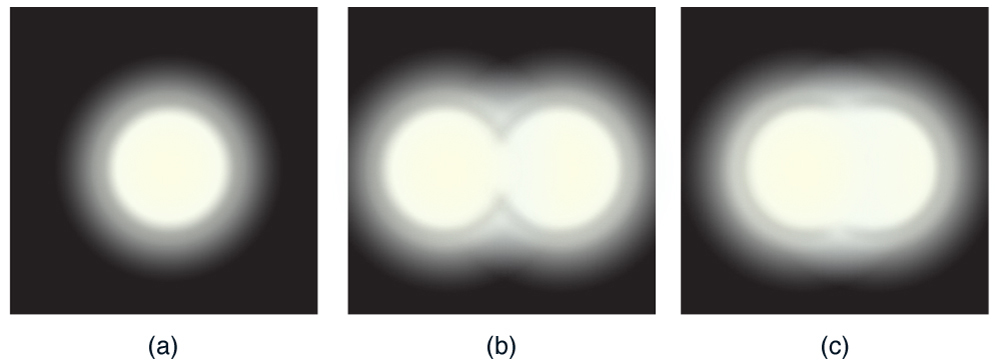The Rayleigh criterion is when two points are just resolved. This is when the central maximum of one image coincides with the first minimum of the other.

The minimum angular separation θ (in radians) for two points to be just resolved is given by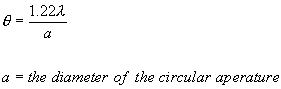where λ is the wavelength and a is the diameter of the circular aperture lens receiving the image (see previous section).

### FYI

Importance of resolution in technology

• CDs and DVDs: By using laser beams with shorter wavelength, we can improve resolving power of the laser and increase the amount of data stored on the discs.
• Electron microscope: Short wavelength of electrons allows electron microscopes to create images with very high resolution.
• Radio telescopes: Radio waves have long wavelengths so the aperture (satellite dish) needs to be very large for a radio telescope to achieve good resolution.

## 9.5 – Doppler effect

• ### The Doppler effect for sound waves and light waves

Doppler equations for sound waves

The Doppler effect refers to the change in observed frequency of a wave due to the movement of the observer and/or that of the wave source.There are four Doppler effect equations for observed frequency depending on different cases:

• Source moving towards observer at rest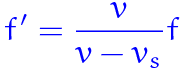• Source moving away from observer at rest• Observer moving towards stationary source• Observer moving away from stationary source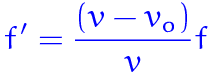where f’ is the observed frequency of the wave emitted by the source and received by the observer, f is the original frequency of the wave, v is the velocity of the wave, and v0 is the velocity of the observer.

 Velocity of the wave Wavelength of the wave Frequency of the wave Moving observer Changes Constant Changes Moving source Constant Changes Changes

Frequency observed changes according to the equation v=fλ.

Doppler equation for electromagnetic waveswhere Δf is the change in frequency of the wave received by the observer as compared to the original frequency emitted by the source, v is the velocity of the observer, c is the speed of light, and f is the original frequency of the wave.

• This equation should only be used when the velocity of the observer is much smaller than the speed of light (v<
• Add Δf to f to obtain the observed frequency (f’) when the wave source and the observer are moving towards each other.
• Subtract Δf from f to obtain the observed frequency (f’) when the wave source and the observer are moving away from each other.

Application of the Doppler effect in speed detectors:

• A beam of electromagnetic wave is fired at the car.
• The frequency of the reflected wave is compared to that of the original wave beam. A higher frequency indicates that the car is moving towards the detector and a lower frequency indicates that the car is moving away from the detector.
• The speed of the car is calculated from the extent of shift in frequency by v=fλ.

Take note that the overall difference in frequency is 2Δf from the equation because the wave travels to the car and then back to the speed detector.